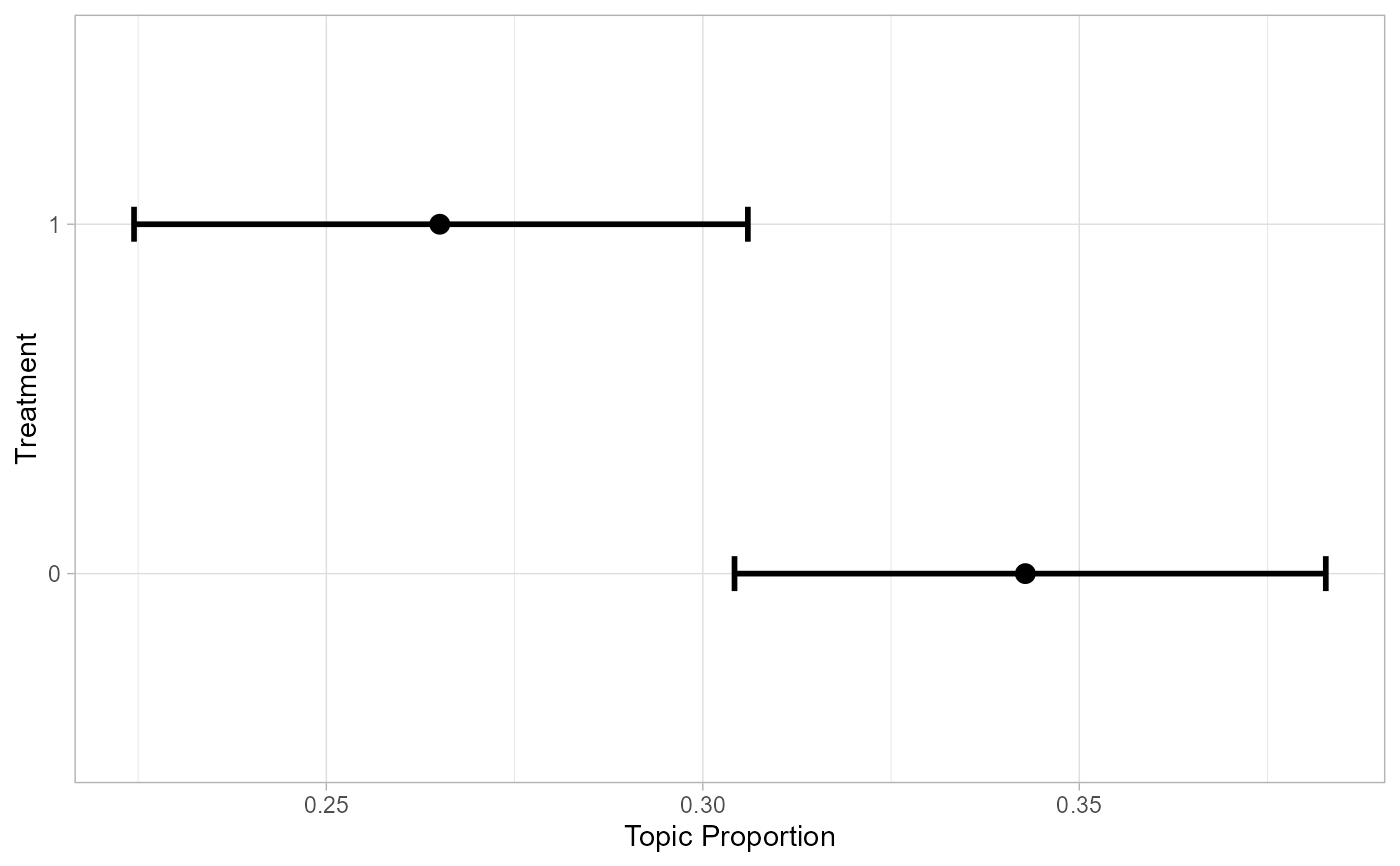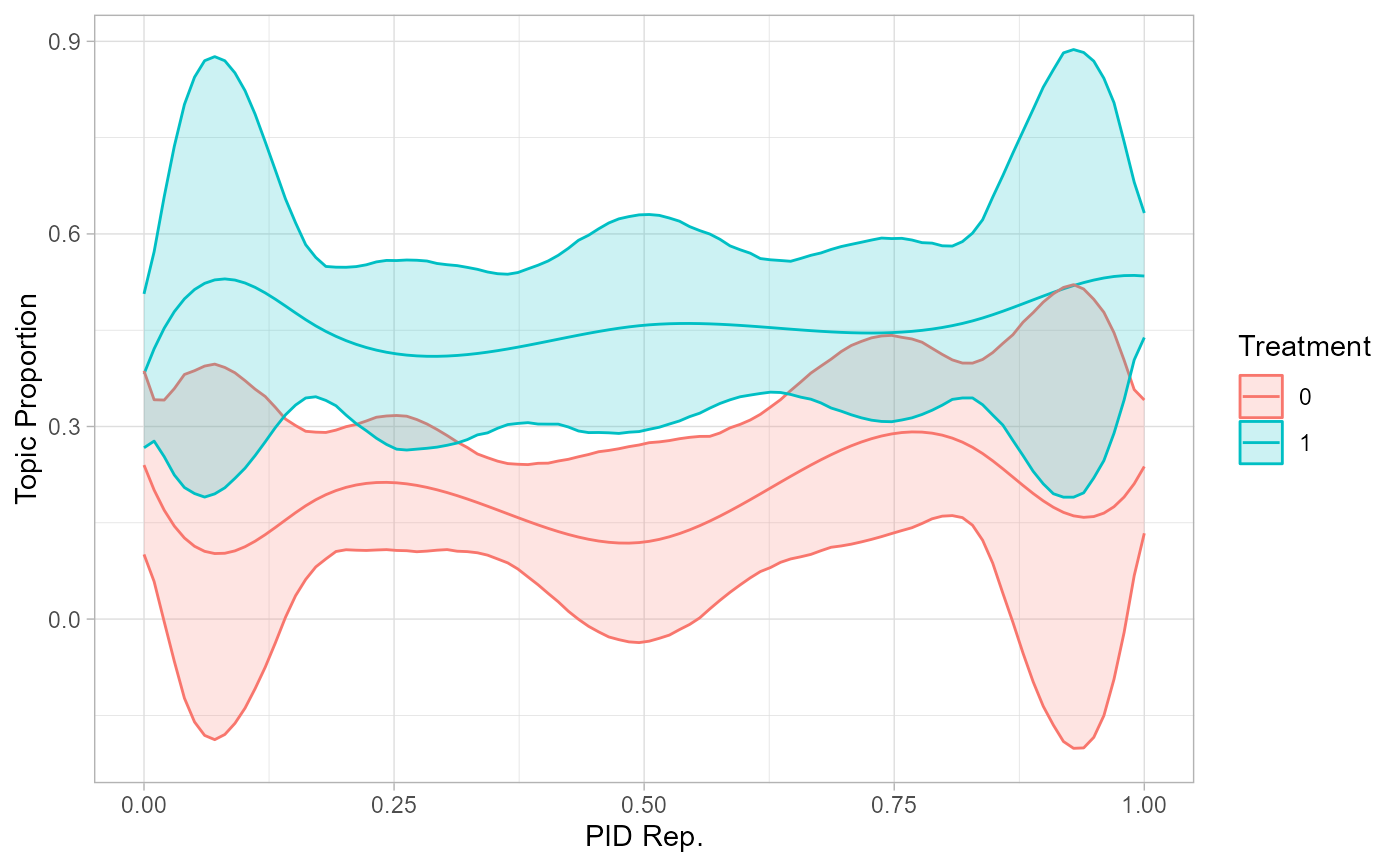get_effects() is a helper function to store effect estimates from stm in a data frame.

get_effects(
estimates,
variable,
type,
ci = 0.95,
moderator = NULL,
modval = NULL,
cov_val1 = NULL,
cov_val2 = NULL
)

## Arguments

estimates The object containing estimates calculated with estimateEffect. The variable for which estimates should be extracted. The estimate type. Must be either 'pointestimate', 'continuous', or 'difference'. The confidence interval for uncertainty estimates. Defaults to 0.95. The moderator variable in case you want to include an interaction effect. The value of the moderator variable for an interaction effect. See examples for combining data for multiple values. The first value of a covariate for type 'difference'. The second value of a covariate for type 'difference'. The topic proportion of 'cov_val2' will be subtracted from the proportion of 'cov_val1'.

## Value

Returns effect estimates in a tidy data frame.

## Examples


library(stm)
library(dplyr)
library(ggplot2)

# store effects

effects <- get_effects(estimates = prep,
variable = 'treatment',
type = 'pointestimate')

# plot effects
effects %>% filter(topic == 3) %>%
ggplot(aes(x = value, y = proportion)) +
geom_errorbar(aes(ymin = lower, ymax = upper), width = 0.1, size = 1) +
geom_point(size = 3) +
coord_flip() + theme_light() + labs(x = 'Treatment', y = 'Topic Proportion')# combine estimates for interaction effects
prep_int <- estimateEffect(1:3 ~ treatment * s(pid_rep),

effects_int <- get_effects(estimates = prep_int,
variable = 'pid_rep',
type = 'continuous',
moderator = 'treatment',
modval = 1) %>%
bind_rows(
get_effects(estimates = prep_int,
variable = 'pid_rep',
type = 'continuous',
moderator = 'treatment',
modval = 0)
)

# plot interaction effects
effects_int %>% filter(topic == 2) %>%
mutate(moderator = as.factor(moderator)) %>%
ggplot(aes(x = value, y = proportion, color = moderator,
group = moderator, fill = moderator)) +
geom_line() +
geom_ribbon(aes(ymin = lower, ymax = upper), alpha = 0.2)  +
theme_light() + labs(x = 'PID Rep.', y = 'Topic Proportion',
color = 'Treatment', group = 'Treatment', fill = 'Treatment')